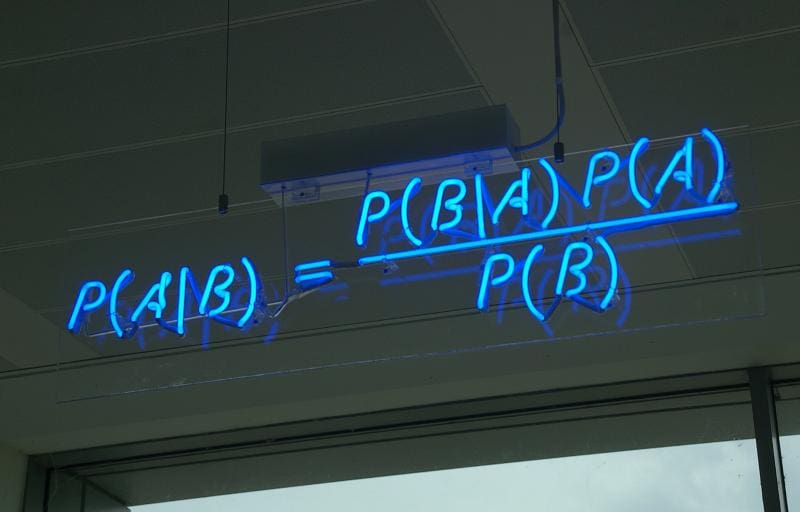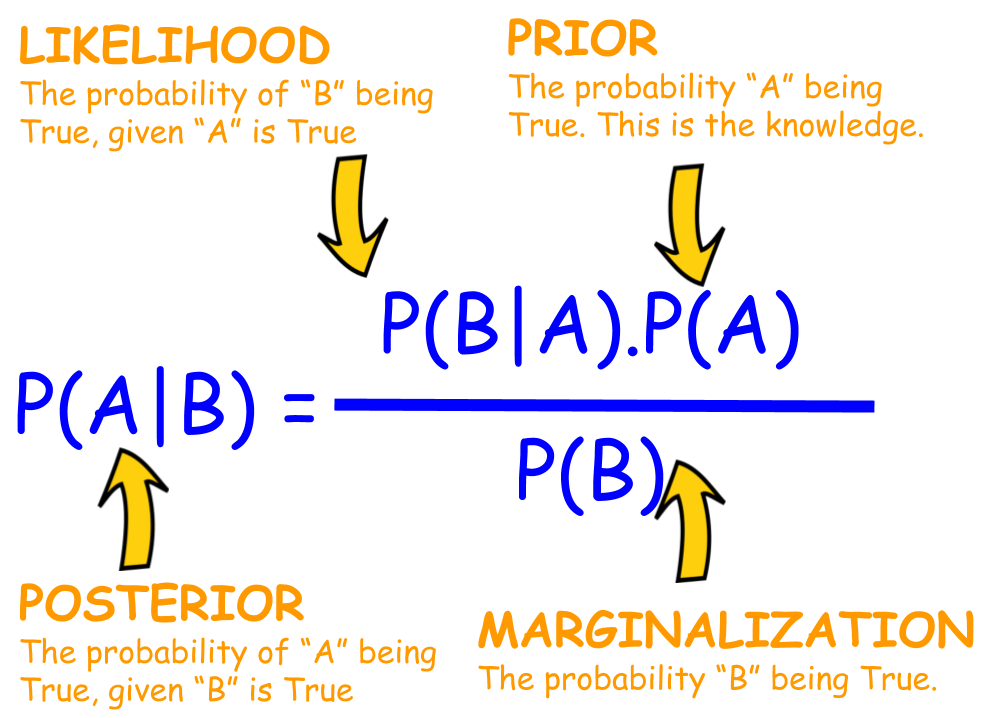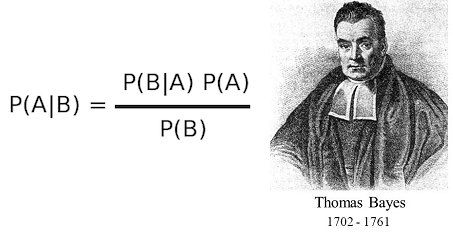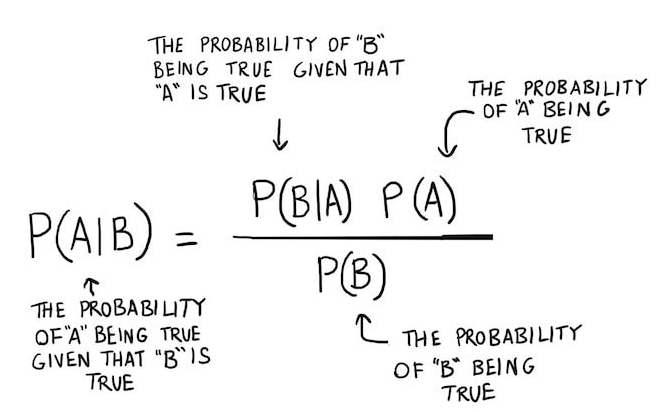1592364240

# Naïve Bayes Algorithm: Everything you need to know

Naïve Bayes is a probabilistic machine learning algorithm based on the Bayes Theorem, used in a wide variety of classification tasks. In this article, we will understand the Naïve Bayes algorithm and all essential concepts so that there is no room for doubts in understanding.
The simplest solutions are usually the most powerful ones, and Naïve Bayes is a good example of that. Despite the advances in Machine Learning in the last years, it has proven to not only be simple but also fast, accurate, and reliable. It has been successfully used for many purposes, but it works particularly well with natural language processing (NLP) problems.

#bayes theorem #machine learning #python

## Buddha Community1596465840

## The Ironic Sophistication of Naive Bayes Classifiers

#### Filtering spam with Multinomial Naive Bayes (From Scratch)

In the first half of 2020 more than 50% of all email traffic on the planet was spam. Spammers typically receive 1 reply for every 12,500,000 emails sent which doesn’t sound like much until you realize more than 15 billion spam emails are being sent each and every day. Spam is costing businesses 20–200 billion dollars per year and that number is only expected to grow.What can we do to save ourselves from spam???

#### Naive Bayes Classifiers

In probability theory and statistics, Bayes’ theorem (alternatively Bayes’s theoremBayes’s law or Bayes’s rule) describes the probability of an event, based on prior knowledge of conditions that might be related to the event.

For example, if the risk of developing health problems is known to increase with age, Bayes’s theorem allows the risk to an individual of a known age to be assessed more accurately than simply assuming that the individual is typical of the population as a whole.Bayes Theorem Explained

#### A Naive Bayes Classifier is a probabilistic classifier that uses Bayes theorem with strong independence (naive) assumptions between features.

• Probabilistic classifier: a classifier that is able to predict, given an observation of an input, a probability distribution over a set of classes, rather than only outputting the most likely class that the observation should belong to.
• Independence: Two events are **independent **if the occurrence of one does not affect the probability of occurrence of the other (equivalently, does not affect the odds). That assumption of independence of features is what makes Naive Bayes naive! In real world, the independence assumption is often violated, but naive Bayes classiﬁers still tend to perform very well.

#naive-bayes-classifier #python #naive-bayes #naive-bayes-from-scratch #naive-bayes-in-python1623085500

## Learn Naive Bayes Algorithm For Machine Learning [With Examples]

### **Introduction  **

In mathematics and programming, some of the simplest solutions are usually the most powerful ones. The naïve Bayes Algorithm comes as a classic example of this statement. Even with the strong and rapid advancement and development in the field of Machine Learning, this Naïve Bayes Algorithm still stands strong as one of the most widely used and efficient algorithms. The naïve Bayes Algorithm finds its applications in a variety of problems including Classification tasks and Natural Language Processing (NLP) problems.

The mathematical hypothesis of the Bayes Theorem serves as the fundamental concept behind this Naïve Bayes Algorithm. In this article, we shall go through the basics of Bayes Theorem, the Naïve Bayes Algorithm along with its implementation in Python with a real-time example problem. Along with these, we shall also look at some advantages and disadvantages of the Naïve Bayes Algorithm in comparison with its competitors.

### **Basics of Probability **

Before we venture out on understanding the Bayes Theorem and Naïve Bayes Algorithm, let us brush up our existing knowledge upon the fundamentals of Probability.

As we all know by definition, given an event A, the probability of that event occurring is given by P(A). In probability, two events A and B are termed as independent events if the occurrence of event A does not alter the probability of occurrence of event B and vice versa. On the other hand, if one’s occurrence changes the probability of the other, then they are termed as Dependent events.

Let us get introduced to a new term called Conditional Probability. In mathematics, Conditional Probability for two events A and B given by P (A| B) is defined as the probability of the occurrence of event A given that event B has already occurred. Depending upon the relationship between the two events A and B as to whether they are dependent or independent, Conditional Probability is calculated in two ways.

• The conditional probability of two dependent events A and B is given by P (A| B) = P (A and B) / P (B)
• The expression for the conditional probability of two independent events A and B is given by, P (A| B) = P (A)

Knowing the math behind Probability and Conditional Probabilities, let us now move on towards the Bayes Theorem.

### **Bayes Theorem **

In statistics and probability theory, the Bayes’ Theorem also known as the Bayes’ rule is used to determine the conditional probability of events. In other words, the Bayes’ theorem describes the probability of an event based on prior knowledge of the conditions that might be relevant to the event.

To understand it in a simpler way, consider that we need to know the probability of the price of a house is very high. If we know about the other parameters such as the presence of schools, medical shops and hospitals nearby, then we can make a more accurate assessment of the same. This is exactly what the Bayes Theorem performs.

Such that,

• P(A|B) – the conditional probability of event A occurring, given event B has occurred also known as Posterior Probability.
• P(B|A) – the conditional probability of event B occurring, given event A has occurred also known as Likelihood Probability.
• P(A) – the probability of event A occurring also known as Prior Probability.
• P(B) – the probability of event B occurring also known as Marginal Probability.

Suppose we have a simple Machine Learning problem with ‘n’ independent variables and the dependent variable which is the output is a Boolean value (True or False). Suppose the independent attributes are categorical in nature let us consider 2 categories for this example. Hence, with these data, we need to calculate the value of the Likelihood Probability, P(B|A).

Hence, on observing the above we find that we need to calculate 2*(2^n -1) parameters in order to learn this Machine Learning model. Similarly, if we have 30 Boolean independent attributes, then the total number of parameters to be calculated will be close to 3 billion which is extremely high in computational cost.

This difficulty in building a Machine Learning model with the Bayes Theorem led to the birth and development of the Naïve Bayes Algorithm.

#artificial intelligence #learn naive bayes algorithm #naive bayes1593865980

## What is the NaïveBayes Algorithm?

Naïve Bayes Algorithm is one of the popular classificationmachine learning algorithms and is included in supervised learning. that helps to classify the data based upon the conditional probability values computation. This algorithm is quite popular to be used in Natural Language Processingor NLP also real-time prediction, multi-class prediction, recommendation system, text classification, and sentiment analysis use cases. the algorithm is scalable and easy to implement for the large data set.Thomas Bayes

The algorithm based on **Bayes theorem. **Bayes Theorem helps us to find the probability of a hypothesis given our prior knowledge.

Let’s look at the equation for Bayes Theorem,Bayes Theorem

Naïve Bayes is a simple but surprisingly powerful predictive modeling algorithm. Naïve Bayes classifier calculates the probabilities for every factor. Then it selects the outcome with the highest probability.

## Applications of Naïve Bayes Algorithm

1. Real-time prediction: Naïve Bayes Algorithm is fast and always ready to learn hence best suited for real-time predictions.

2. Multi-class prediction: The probability of multi-classes of any target variable can be predicted using a Naïve Bayes algorithm.

3. Text Classification where Naïve Bayes is mostly used is Spam Filtering in Emails (Naïve Bayes is widely used for text classification)

4. Text classification/ Sentiment Analysis/ Spam Filtering: Due to its better performance with multi-class problems and its independence rule, Naïve Bayes algorithm perform better or have a higher success rate in text classification, Therefore, it is used in Sentiment Analysis and Spam filtering.

5.**Recommendation System: **Naïve Bayes Classifier and Collaborative Filtering together build a Recommendation System that uses machine learning and data mining techniques to filter unseen information and predict whether a user would like a given resource or not.

#data-driven-investor #data-science #naive-bayes-classifier #machine-learning #python #naïve bayes1592364240

## Naïve Bayes Algorithm: Everything you need to know

Naïve Bayes is a probabilistic machine learning algorithm based on the Bayes Theorem, used in a wide variety of classification tasks. In this article, we will understand the Naïve Bayes algorithm and all essential concepts so that there is no room for doubts in understanding.
The simplest solutions are usually the most powerful ones, and Naïve Bayes is a good example of that. Despite the advances in Machine Learning in the last years, it has proven to not only be simple but also fast, accurate, and reliable. It has been successfully used for many purposes, but it works particularly well with natural language processing (NLP) problems.

#bayes theorem #machine learning #python1624955640

## Social Media Algorithms: Everything You Need to Know

• Social media platforms employ algorithms backed by Artificial Intelligence and Machine Learning
• The algorithms use relevancy and consumer behaviour to dictate social feeds
• Content creators must strategize to achieve quality interactions from users and work with the algorithm, not against it

There are nearly 4 billion internet users, out of which 3 billion have a social media presence. The magnitude of data transmitted every minute or every second is incomprehensible. But there’s a method by which social media platforms maintains order, sorts this data, and ranks it. That’s what a social media algorithm does.

### What are Social Media Algorithms?

They are mathematical rules that dictate how a user will see content on their social media account. They sort posts on the feed basis the relevancy of the published content to a specific user, ascertained by user behavior. They want traffic to flow into their platforms continually, and therefore, relevancy gains precedence over chronological order.

### No Two Platforms Use the Same Algorithm

Read on to find out how do social media algorithms work on different platforms.

• Social media platforms employ algorithms backed by Artificial Intelligence and Machine Learning
• The algorithms use relevancy and consumer behaviour to dictate social feeds
• Content creators must strategize to achieve quality interactions from users and work with the algorithm, not against it

There are nearly 4 billion internet users, out of which 3 billion have a social media presence. The magnitude of data transmitted every minute or every second is incomprehensible. But there’s a method by which social media platforms maintains order, sorts this data, and ranks it. That’s what a social media algorithm does.

### What are Social Media Algorithms?

They are mathematical rules that dictate how a user will see content on their social media account. They sort posts on the feed basis the relevancy of the published content to a specific user, ascertained by user behavior. They want traffic to flow into their platforms continually, and therefore, relevancy gains precedence over chronological order.

### No Two Platforms Use the Same Algorithm

Read on to find out how do social media algorithms work on different platforms.